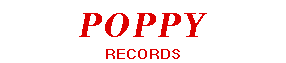WAX CYLINDER GEOMETRY

The description which follows applies to standard 'Edison' cylinders, normally called "2-minute cylinders". Figures for '4-minute' cylinders are given in red. There are many other types of cylinder for which the dimensions shown below may not be appropriate.

The dimensions of wax will change significantly with temperature, so an appropriate temperature correction must be applied when measuring the dimensions of a cylinder in practice.

CYLINDER BORE DIMENSIONS

The standard cylinder bore is a truncated cone with a taper of 3/8" per foot (1:32) and a length of about 4" . The largest diameter of the bore should be around 113/16" (1·875") and the smallest around 15/8" (1·6875") but the mandrel should be longer than 4" and run over a greater range of diameters to allow for oversized and undersized cylinders which may locate themselves nearer the larger or the smaller diameter ends.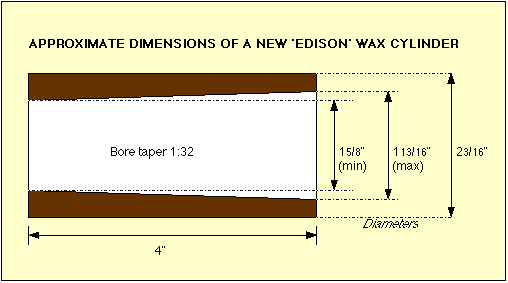The outer diameter of a new cylinder is approximately 23/16" but this will be reduced each time shaving and re-recording is carried out. The inside of the bore may be ribbed or lined; but in making a mandrel, the above dimensions may be assumed to give a suitable locating surface.

GROOVE SPIRAL

The groove is in the form of a spiral of 100 (200) turns per longitudinal inch. The spiral is 'handed' such that when a horizontally-mounted cylinder is rotated such that the top moves towards the observer, the spiral tracks from right to left. The start is at the larger bore end.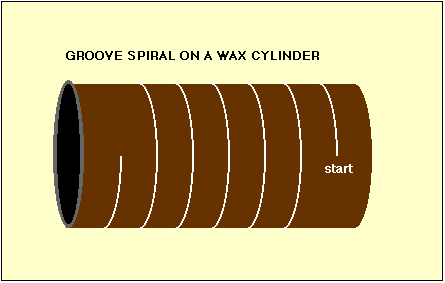The recording speed varied greatly between different types and makes of cylinders. If there is a 'standard' speed, it is probably 160 rpm for commercial entertainment cylinders and 100 rpm for home and office recording.

GROOVE DIMENSIONS

The groove is cut with a tool made from a cylindrical sapphire rod, cut and polished to form a cutting facet at an angle of 15 degrees to the longitudinal axis. It is mounted, as shown below, to produce an angle between the cutting facet and the normal of the wax surface of 10 degrees.

The sapphire rods are standard components from the Swiss watch-making industry and have a diameter of 0.9 (0.6) mm. or 0·0354" (0·0236")The relationship between the groove width and depth of cut can be calculated by simple geometry, provided the cutting facet is assumed to produce a groove which is a segment of a circle. This is not quite a correct assumption, because the cutting tool is angled to the wax surface, which produces a slightly elliptical groove cross section. For all practical purposes, however, a circular shape can be assumed.

The diagram below shows a shallow segmental groove cut in wax by a circular cutting facet
where:

 C is the centre of the 'circular' cutting facet of radius r B is the width of the cut groove A is the depth of cutTo calculate A from B and r Pythagoras r^2 = s^2 + (B/2)^2 Where ^ indicates "to the power of" (1) So s^2 = r^2 - (B/2)^2 (2) Now A = r - s (3) Therefore A = r - Sqrt(r^2 - (B/2)^2 ) (4)

If any possibility of cutting into a previous turn is to be avoided, the maximum depth of cut is limited by the maximum allowable width of the groove. As the turns are spaced at 100 (200) per inch, the maximum width (B) is limited to 0·01" (0·005").

The maximum depth of cut is therefore:

 2-minute 4-minute Max width (B) 0·01" 0·005" Cutter dia (2r) 0·0354" 0·0236" r = 0·0177" 0·0118" From Equn (4)   A = Depth at which intercutting between adjacent turns occurs 0·000721" 0·000268"

MODULATION DEPTH

The cutter is modulated at right angles to the wax surface ("hill & dale" or "vertical" modulation) and it is assumed that the modulation is symmetrical about the mean cutter depth. In this case, the minimum grooove depth is zero and the maximum is determined by the intercutting depth calculated above.

Therefore an unmodulated groove will have to be cut at half the intercutting depth, if intercutting on peaks of modulation is to be completely avoided. In practice, a slightly deeper cut than the theoretically correct one may be employed and the risk of a certain amount of groove inter-cutting tolerated. Many commercial cylinders show signs of inter-cutting.

The maximum modulation (peak-to-peak) will be the same as the intercutting depth.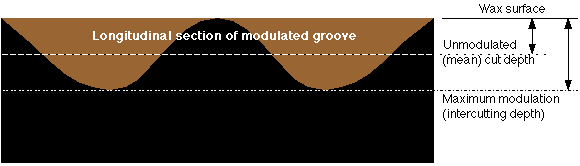SLEW RATE LIMIT

When the cutting tip is following the downwards portion of the modulation waveform (increasing cutting depth), there should be clearance between the back of the cutter and the already-cut groove.At high slew rates there is the risk that the back of the cutting tool will contact the groove it has just cut and damage it. This sets a limit to the maximum slew rate and hence the maximum amplitude of modulation at a given frequency.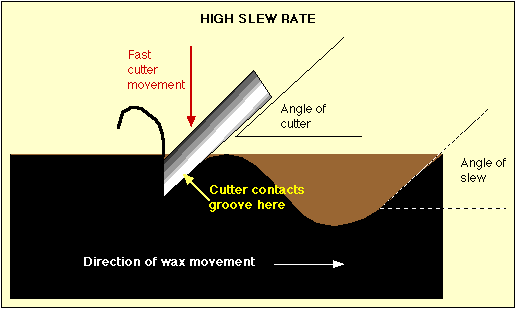To calculate the maximum slew rate, the speed of the wax past the cutter must be known. A figure of 2" dia can be taken as representative of a part-worn cylinder. At 160 rpm this represents a surface speed of:

 (160 / 60) x 2 x pi inches per second = 16·76 ips

The 'back angle' of the cutter is about 5 degrees, so the maximum downwards speed of the cutter is:

 16·76 x Tan5 ips = 1·466 ips (5)

From this it is possible to calculate the maximum frequency (fms) at which full amplitude modulation may be used.

 Peak-peak modulation amplitude (ap-p) = 0·000721"  0·000268" Peak modulation velocity (v) = ap-p x 2 x pi x fms fms =  v / (ap-p x 2 x pi) From Eqn (5) v = 1·466 so  fms =  324Hz  871 Hz
[Note: For 100 rpm cylinders at 100 tpi only, the figure will be 202 Hz]

Above fms, a means of decreasing the signal amplitude at the rate of 6dB per octave must be interposed between the signal source and the cutter to prevent slew-rate distortion. If the turnover frequency of the 'Blumlein' recording equalisation characteristic is at (or below) fms, the 'constant velocity' portion of the characteristic will produce the necessary amplitude reduction and additional equalisation will not be needed.

RECORDED VELOCITY

From Eqn (5) [above] the peak modulation velocity (v) is 1·466 ips. Recording engineers are more familiar with metric units, so this is equivalent to 3·724 cm/sec peak or 2·63 cm/s RMS

ACCELERATION

Acceleration (s) can be calculated from velocity (v) and frequency (f):

 s = v x 2 pi f (6)

At 1 kHz, the maximum modulation of a 100 tpi recording is limited by slew rate, not by amplitude.

 Maximum RMS velocity v = 2·63 cm/s Relative to 1 cm/s =  8·4 dBCALIBRATION

CALIBRATION AT 1 KHZ

Calibration of an electrically driven cutterhead is possible without access to sophisticated instruments by using the acceleration due to gravity as a starting point.

 Acceleration due to gravity (g) = 981 cm/sec/sec From (6) v = s / ( 2 pi f ) At a frequency of 1 kHz v = 981 / ( 2 pi x 1000 ) v = 0·1561 cm/s

The cutterhead should be mounted with the stylus at the top so that the modulation direction is vertical and arranged so that a small hard object can be loosely resting on the cutter.

The cutter is driven with a sinewave at 1 kHz and the amplitude gradually increased until the object is found to lose contact with the cutter during part of the cycle. At that point the peak velocity will have just exceeded 0.1561 cm/s. The test is only moderately accurate and should be repeated several time to obtain an average value.

To obtain a reference modulation of 1 cm/s RMS at 1 kHz, the drive voltage should be increased by a factor of: (Sqrt 2) / 0·1561 = 9·06 = (19·1 dB)

ALTERNATIVE CALIBRATION POINT

 Acceleration due to gravity (g) = 981 cm/sec/sec From (6) v = s / ( 2 pi f ) f = s / ( 2 pi v ) Frequency for 1cm/s pk = 981 / ( 2 pi x 1 ) Frequency for 1cm/s RMS f cal = 981 / (2 pi x Sqrt 2) f cal = 110·4 Hz

At a frequency of 110·4 Hz, calibration by gravity will give a velocity of 1 cm/s RMS.